# Intermediate Geometry : How to find if of acute / obtuse isosceles triangle are similar

## Example Questions

### Example Question #25 : Triangles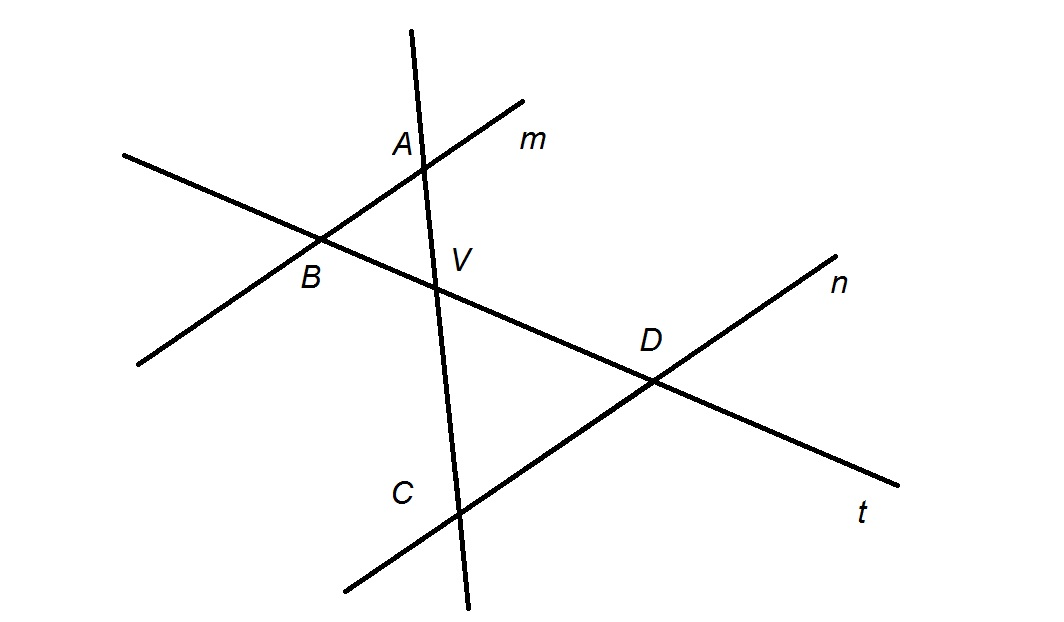Refer to the above diagram.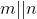.

True or false: From the information given, it follows that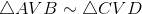.

False

True

True

Explanation:

By the Angle-Angle Similarity Postulate, if two pairs of corresponding angles of a triangle are congruent, the triangles themselves are similar.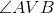and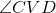are a pair of vertical angles, having the same vertex and having sides opposite each other. As such,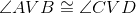.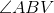and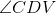are alternating interior angles formed by two parallel linesandcut by a transversal. As a consequence,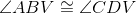.

The conditions of the Angle-Angle Similarity Postulate are satisfied, and it holds that.

### Example Question #26 : TrianglesRefer to the above diagram.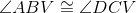.

True or false: From the information given, it follows that.

False

True

False

Explanation:

The given information is actually inconclusive.

By the Angle-Angle Similarity Postulate, if two pairs of corresponding angles of a triangle are congruent, the triangles themselves are similar. Therefore, we seek to prove two of the following three angle congruence statements: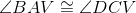andare a pair of vertical angles, having the same vertex and having sides opposite each other. As such,, but this is not one of the statements we need to prove. Also, without further information - for example, whetherandare parallel, which is not given to us - we have no way to prove either of the other two necessary statements.

The correct response is "false".

### Example Question #27 : Triangles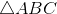and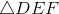are both isosceles triangles;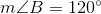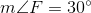True or false: from the given information, it follows that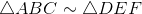.

True

False

False

Explanation:

As we are establishing whether or not, then, andcorrespond respectively to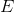, and.is an isosceles triangle, so it must have two congruent angles.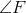has measure, so either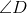has this measure,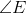has this measure, or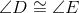. If we examine the second case, it immediately follows that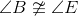. One condition of the similarity of triangles is that all pairs of corresponding angles be congruent; since there is at least one case that violates this condition, it does not necessarily follow that. This makes the correct response "false".

### Example Question #1 : How To Find If Of Acute / Obtuse Isosceles Triangle Are Similaris an equilateral triangle;is an equiangular triangle.

True or false: From the given information, it follows that.

True

False

True

Explanation:

As we are establishing whether or not, then, andcorrespond respectively to, and.

A triangle is equilateral (having three sides of the same length) if and only if it is also equiangular (having three angles of the same measure, each of which is). It follows that all angles of both triangles measure.

Specifically,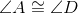and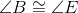, making two pairs of corresponding angles congruent. By the Angle-Angle Similarity Postulate, it follows that, making the correct answer "true".

### Example Question #2 : How To Find If Of Acute / Obtuse Isosceles Triangle Are Similar

Given:andsuch that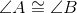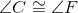Which statement(s) must be true?

(a)(b)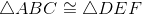(a) but not (b)

(b) but not (a)

(a) and (b)

Neither (a) nor (b)

(a) but not (b)

Explanation:

The sum of the measures of the interior angles of a triangle is, so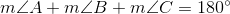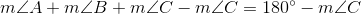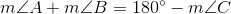Also,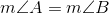,

so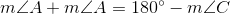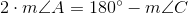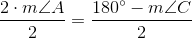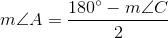By similar reasoning, it holds that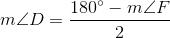Since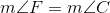, by substitution,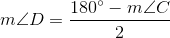Therefore,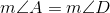,

orThis, along with the statement that, sets up the conditions of the Angle-Angle Similarity Postulate - if two angles of one triangle are congruent to the two corresponding angles of another triangle, the two triangles are similar. It follows that.

However, congruence cannot be proved, since at least one side congruence is needed to prove this. This is not given in the problem.

Therefore, statement (a) must hold, but not necessarily statement (b).